AEPA Math: Solving Trigonometric Equations Chapter Exam

Exam Instructions:

Choose your answers to the questions and click 'Next' to see the next set of questions. You can skip questions if you would like and come back to them later with the yellow "Go To First Skipped Question" button. When you have completed the practice exam, a green submit button will appear. Click it to see your results. Good luck!

Page 1

Question 3 3. Solve the following equation for θ.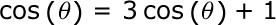Page 4

Question 16 16. Solve the following equation for x.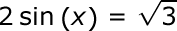Question 19 19. Solve the following trigonometric equation for θ.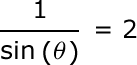Question 20 20. Solve for the value of x in the trigonometric equation below. (Use the domain -π/2 to π/2)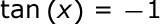Page 5

Question 25 25. Solve the following trigonometric equation for x.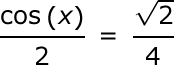AEPA Math: Solving Trigonometric Equations Chapter Exam Instructions

Choose your answers to the questions and click 'Next' to see the next set of questions. You can skip questions if you would like and come back to them later with the yellow "Go To First Skipped Question" button. When you have completed the practice exam, a green submit button will appear. Click it to see your results. Good luck!

Support